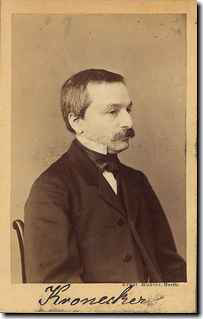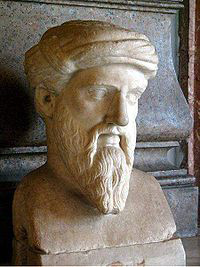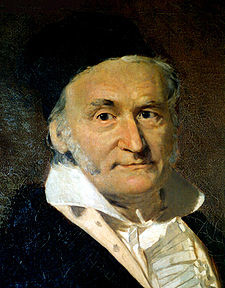# Marys Medicine

## Abelprisen.noAbel Prize 2010
Number Theory; the
ground of John Tate
Algebraic integers, finite fields, p-adic numbers. Class field theory, rigid analytic spaces,
elliptic curves. These are some of the concepts you must know if your task is to describe
the mathematical achievements of John Torrence Tate, the Abel Laureate 2010.
If you don' t know anything about any of the listed words, you can still share something
with one of the most brilliant scientist of our time; the fascination for the natural num-
bers. At the very first glance, they look innocent and easily accessible. Counting, 1, 2, 3,
you realize that the world you are diving into is huge, mysteriuos and unpredictable.
"Mathematics is the queen of the sciences and
number theory is the queen of mathematics."
Carl Friedrich Gauss "Number is the
within of all
Pythagoras of Samos "God invented the integers; all
else is the work of man."
Leopold Kronecker Abel Prize 2010
Number theory is the study of the proper-
ties of numbers in general, and integers in
particular. Number theory may be subdi-
vided into several fields, according to the
methods used and the type of questions
Elementary number theory
In elementary number theory, integers are studied without use of techniques from other mathematical fields. Important discoveries of this field are Fermat's little theorem, Euler's theorem, the Chinese remain- Algebraic number theory
der theorem and the law of quadratic reciprocity, to In algebraic number theory, the concept of a number mention a few.
is expanded to algebraic numbers, i.e. roots of pol- ynomials with rational coefficients. These domains Analytic number theory
contain elements analogous to the integers, the so- Analytic number theory employs the machinery of called algebraic integers, which is a main subject of calculus and complex analysis to tackle questions study in this field. about integers. Examples include the prime number Many number theoretic questions are attacked by theorem concerning the asymptotic behavior of the reduction modulo p for various primes p. This lo- primes and the Riemann hypothesis, but also proofs calization procedure leads to the construction of the of the transcendence of π or e, are classified as ana- p-adic numbers, another main subject of study in lytical number theory. the field of algebraic number theory.
Arithmetic algebraic geometry
Arithmetic (algebraic) geometry is the study of schemes of finite type over the spectrum of the ring of integers Z.
Diophantine geometry is the study of algebraic vari- eties over number fields.
John Tates investigations Combinatorial number theory
mainly belong to the subfield Combinatorial number theory deals with number the- Algebraic number theory.
oretic problems which involve combinatorial ideas in their formulations or solutions. Paul Erdös is the main founder of this branch of number theory. Exam- ples are the problems of finding arithmetic progres- sions in a set of integers.
Other subfields of number Modular forms are analytic functions on the upper half-plane satisfying a certain kind of functional equation and a growth condition. The theory of mod- ular forms therefore belongs to complex analysis but the main importance of the theory has traditionally been in its connections with number theory. Abel Prize 2010
John Tate´s influence in modern number
theory can be read out of the numerous
results and concepts named after him.
Here are some of them:
Hodge-Tate theory; p-adic analogue of the Hodge
decomposition for complex cohomology.
Tate module; a Galois module constructed
from an abelian variety over a field The Lubin–Tate formal group law is the
unique (1-dimensional) formal group law F such that e(x) = px + xp is an endomorphism of F, i.e. such that e(F(x,y)) = F(e(x), e(y)) The Tate Isogeny theorem says that abelian varie-
ties with isomorphic Tate modules are isogenous.
The Sato–Tate conjecture is a statistical state-
ment about the family of elliptic curves E over the finite field with p elements, with p a prime number, obtained from an elliptic curve E over the rational Tate twist; a particular abelian group with an
number field, by the process of reduction modulo a action of a Galois group constructed from a prime for almost all p.
In the theory of elliptic curves, Tate's algo-
Tate motive is the tensor inverse of the Lefschetz
rithm, takes as input an integral model of an
elliptic curve E over Q and a prime p. It returns
the exponent f of p in the conductor of E, the type of reduction at p, and the local index c .p The Tate-Shafarevich group, named for Tate
and Igor Shafarevich, of an abelian variety de- Via the Serre-Tate theorem one can control (part
fined over a number field K consists of the ele- of) the char p deformations of an abelian scheme ments of the Weil-Châtelet group that become coming from the local part of the Barsotti-Tate trivial in all of the completions of K Barsotti-Tate groups; arise in "nature"
when one consider the sequence of kernels Néron–Tate height (or canonical height) is a quad-
of multiplication by successive powers of p ratic form on the Mordell-Weil group of rational on an abelian variety.
points of an abelian variety defined over a global Tate cohomology groups are a slightly modified
form of the usual cohomology groups of a finite group that combine homology and cohomology groups into one sequence.
Honda-Tate theory; i.e. classification of abelian
varieties over finite fields up to isogeny.
Abel Prize 2010
A fundamental result in number theory
is the Unique-Prime-Factorization Theo-
rem for integers. In 1847, in an attempt
to prove Fermats Last Theorem, Gabriel
Lamé incorrectly assumed that this prop-
erty holds in general. He was immediate-
ly corrected by Joseph Liouville who re-
The Fundamental Theorem of Arithmetic
ferred to results of Ernst Kummer about
The Fundamental Theorem of Arithmetic (or failure of unique prime factorization in
Unique-Prime-Factorization Theorem) states certain rings of algebraic integers, pub-
that any integer greater than 1 can be written as a lished in 1843.
unique product (up to ordering of the factors) of This innocent little dispute became the
prime numbers. Intuitively, this theorem character- origin of a branch of number theory, in
izes prime numbers uniquely in the sense that they are the "fundamental numbers." The theorem was which John Tate has been a main figure
practically proved by Euclid but the first full and during the last 50 years.
correct proof is found in the Disquisitiones Arith- meticae by Carl Friedrich Gauss.
Prime factorization in
algebraic number fields
A fundamental property of the integers is the unique In number theory the starting points is the set of in- factorization property. There is only on way of writ- tegers, ., -3, -2, -1, 0, 1, 2, 3, ., denoted by Z. The
ing 105 as a product of primes (105=3·5·7) when we integers are included in the rational numbers Q, i.e.
do not bother about the order of the factors. But in all fractions of integers, where the denominator is a general algebraic number field this is no longer different from 0. Unfortunately the number �2, de- true. The favourite example for (nearly) all math- fined as the root of the polynomial equation x2-2=0 is ematicians is the extension of Q by the square root
not included in Q, as observed by the Pythagoreans
of -5. (If you have bad feelings for the square root around 400 BC. Nevertheless, we are interested in of a negative number, just close your eyes and keep studying the properties of �2, so we extend Q by �2
walking. You will get used to it.) In this extension, to obtain our first homemade algebraic number field, or rather the integral part of it, the number 6 has two denoted Q(�2), consisting of all number which can
different prime factorizations.
be written as a+b�2, for rational numbers a and b.
6=2·3=(1+ �-5)·(1- �-5) The number �2 is defined as the solution of certain All factors involved are prime numbers, i.e. only di- polynomial equation. One can in fact show that all visible by 1 and itself. numbers in Q(�2) satisfy some polynomial equation.
The extent to which unique factorization fails in the Not the same one for all, but at least one for each. ring of integers of an algebraic number field can be Some rational numbers are integers, and some of the described by a certain group known as a class group. algebraic numbers are algebraic integers. The way If the class group is finite, then the order of the group we decide if a number should be called an integer, is is called the class number. The class group of the as follows; we look at the monic polynomial equa- algebraic integers of an algebraic number field is tion for the number (monic means that the coefficient trivial if and only if the ring of algebraic integers of the highest degree term is 1). If all the coefficients has the unique factorization property. The size of the are integers, all roots of the equation are algebraic ideal class group can thus be considered as a meas- integers, If not, they are not! Examples of algebraic ure for the deviation from being a unique factoriza- integers in Q(�2) are �2 (root of the polynomial x2-
tion domain.
2) and 1+�2 (root of x2-2x-1).

Source: http://www.abelprisen.no/nedlastning/2010/tate_en.pdf

### Report cover - forest environment

Nga Aho Rangahau o Maniapoto Forest opportunities Barbara Hock, Marie Heaphy, Duncan Harrison and Tim Payn REPORT INFORMATION SHEET REPORT TITLE NGA AHO RANGAHAU O MANIAPOTO: FOREST OPPORTUNITIES AUTHORS BARBARA HOCK, MARIE HEAPHY, DUNCAN HARRISON, AND TIM MANIAPOTO MĀORI TRUST BOARD CLIENT CONTRACT NO:

### 5.xps

African Journal of Basic & Applied Sciences 2 (1-2): 25-29, 2010 © IDOSI Publications, 2010 Synergistic Effect of Salvadora persica Extracts, Tetracycline and Penicillin Against Staphylococcus aureus 1Zafar Ahmed, S 1 haukat Saeed Khan, M ahnaaz Khan, 1Arshiya Tanveer and Zah oor Ahmad Lone